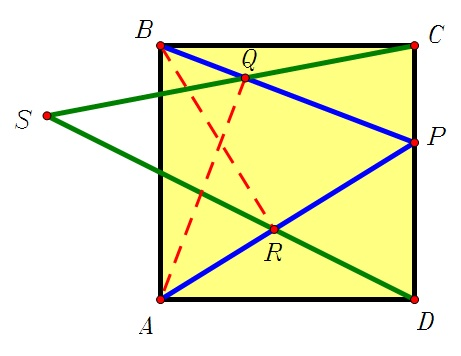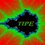# A square and some other pointsLet $ABCD$ be a square and $P$ be a point on the side $CD$ ($P\neq C$ and $P\neq D$ ). Let $AQ$ and $BR$ be heights in the triangle $ABP$, and let $S$ be the interesection point of lines $CQ$ and $DR$. Prove that $\angle ASB=90°$.Note by Jorge Tipe
7 years, 4 months ago

This discussion board is a place to discuss our Daily Challenges and the math and science related to those challenges. Explanations are more than just a solution — they should explain the steps and thinking strategies that you used to obtain the solution. Comments should further the discussion of math and science.

When posting on Brilliant:

• Use the emojis to react to an explanation, whether you're congratulating a job well done , or just really confused .
• Ask specific questions about the challenge or the steps in somebody's explanation. Well-posed questions can add a lot to the discussion, but posting "I don't understand!" doesn't help anyone.
• Try to contribute something new to the discussion, whether it is an extension, generalization or other idea related to the challenge.
• Stay on topic — we're all here to learn more about math and science, not to hear about your favorite get-rich-quick scheme or current world events.

MarkdownAppears as
*italics* or _italics_ italics
**bold** or __bold__ bold
- bulleted- list
• bulleted
• list
1. numbered2. list
1. numbered
2. list
Note: you must add a full line of space before and after lists for them to show up correctly
paragraph 1paragraph 2

paragraph 1

paragraph 2

[example link](https://brilliant.org)example link
> This is a quote
This is a quote
    # I indented these lines
# 4 spaces, and now they show
# up as a code block.

print "hello world"
# I indented these lines
# 4 spaces, and now they show
# up as a code block.

print "hello world"
MathAppears as
Remember to wrap math in $$ ... $$ or $ ... $ to ensure proper formatting.
2 \times 3 $2 \times 3$
2^{34} $2^{34}$
a_{i-1} $a_{i-1}$
\frac{2}{3} $\frac{2}{3}$
\sqrt{2} $\sqrt{2}$
\sum_{i=1}^3 $\sum_{i=1}^3$
\sin \theta $\sin \theta$
\boxed{123} $\boxed{123}$

## Comments

Sort by:

Top Newest

I will give you some hints. 1. Suppose line $AQ$ intersect side $BC$ at $M$, and line $BR$ intersect side $AD$ at $N$. Then $QMCP$ and $RPDN$ are cyclic. 2. In the picture there are many pairs of congruent triangles.

- 7 years, 4 months ago

Log in to reply

Thanks Jorge I know about the congruent triangles and I think I can proceed from your first hint to prove the statement in the problem

I am still struggling to find a solution to the least value of z satisfying z^3=a^4+b^4+(a+b)^4 for distinct positive integers a and b

All I want is the answer not the solution

Can you help me

- 7 years, 4 months ago

Log in to reply

The answer is 392.

- 7 years, 4 months ago

Log in to reply

Many thanks Jorge

Will not trouble you again

Good luck with the Olympiad training

Des O Carroll

- 7 years, 4 months ago

Log in to reply

presumably we can let p be the midpoint of cd and use coordinate geometry

the calculations turn out to be a little awkward as is usual when using this method but it is the only way I can prove the result would love to see other solutions

- 7 years, 4 months ago

Log in to reply

Sir what if we draw a circle and we use tangent properties to prove the problem

- 4 years, 4 months ago

Log in to reply

Jorge's hint was what I first thought of to trigger some cyclics , however I will present another proof(more or less the same but probably differ in motivation since one is more of the wishful thinking type and one is more direct and logical)

It suffices to prove $\angle CQP+\angle PRD=90$, this inspired me to drop pedals from $C,D$ to $PB,PA$ respectively, denote them $X,Y$, now we just have to prove $\triangle CQX\sim \triangle RDY\iff \frac {CX}{QX}=\frac {RY}{DY}$. Now pay some attention to congruent$\triangle BCX,\triangle ABQ$ and $\triangle ADX, \triangle BAR$ and the rest is not hard and can be proceeded multiple ways.

- 7 years, 3 months ago

Log in to reply

in triangle abc angle a+b=90°, c=?

- 6 years, 8 months ago

Log in to reply

×

Problem Loading...

Note Loading...

Set Loading...